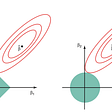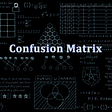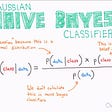# IMPROVE MACHINE LEARNING MODEL PERFORMANCE -with Hyper-parameter tuning

Or you missing some thing when you implement logistics regression.

Or if you want to improve performance of your logistic regression.

Don’t worry you are on Right place.

We will cover all these topics ..

1. Implement logistics regression with some random parameter.
2. Then we will check the accuracy with default parameter.
3. We will try to improve accuracy of logistics regression using hyper-parameter tuning.
4. After apply hyper parameter tuning we will check the accuracy once again.

This dataset is already cleaned. so no need of preprocessing , feature engineering , feature extractions and all on it.

Dataset look like-

So here we go -

Step 1-

`import pandas as pdimport numpy as npda=pd.read_csv('FileName.csv')`

Importing necessary libraries and dataset.

Step 2-

`from sklearn.preprocessing import MinMaxScalerscaler = MinMaxScaler()da[['Age','Fare']]=scaler.fit_transform(da[['Age','Fare']].values)`

Performing little bit feature scaling mix-max scale on numeric data.

(Age),(Fare).

After performing feature scaling on data.

Step 3-

`x=da['Survived']y=da.iloc[:,1:8]`

Divide the dataset into dependent or independent features for further processing.

Step 4-

`from sklearn.linear_model import LogisticRegressionfrom sklearn.linear_model import LogisticRegressionfrom sklearn.model_selection import train_test_split from sklearn import metrics`

Import all libraries which will be useful for implement logistics regression , spilte data into train , test and check the accuracy of the model.

Step 5-

`ourmodel = LogisticRegression(C=0.01,solver='liblinear' )`

initialize logistics regression with some random parameter.

C value is 0.01.

Or solver =”liblinear”.

Step -6

`X_train, X_test, y_train, y_test = train_test_split(y, x, test_size=0.2, random_state=0)`

Spilte dataset into train and test .

80 % for training or 20% for testing , random state =0.

Step 7-

`ourmodel.fit(X_train, y_train)`

Fit the dataset for training.

Step 8-

`y_pred = ourmodel.predict(X_test) # here is prediction`

Find prediction of our test dataset and then measure the accuracy of the model.

Step 9-

`accuracy = metrics.accuracy_score(y_test, y_pred)print('Accuracy: {:.2f}'.format(accuracy))`

Our model is giving 66% accuracy .which is not good.

So that our model performing worst.

How can improve performance of our model.

Now for improving model performance we will use hyper-parameter tuning on logistics regression .

For performing hyper-parameter tuning on logistics regression . we will use this time grid search.

step 10-

If you do not know about grid search click on this link-https://towardsdatascience.com/random-search-vs-grid-search-for-hyperparameter-optimization-345e1422899d

`from sklearn.model_selection import GridSearchCVparam_grid = {'C': [  10, 100,1.0,0.1,0.01],'solver': ['newton-cg','lbfgs','liblinear'],'penalty': ['l2']}grid = GridSearchCV(LogisticRegression(), param_grid, refit = True, verbose = 3)grid.fit(X_train, y_train)`

Importing grid search for searching best parameter for our model.

Initialize the grid search with -

In first parameter - write of your machine learning model name.Which you want to use.

In second parameter-pass the dictionary with different-2 parameter. The grid search will apply permutations and combination with different-2 parameter.then it give best combinations of parameters.

Set refit =true , for fit different-2 combination again and again.

after Initialize grid search . we are going to fit the training data in it.

Step 11-

`print(grid.best_params_)`

grid.best_params — give best parameters of our model.

It giving best parameter of the model.

once again , when we will initialize the model with these parameters .our model will give 83% accuracy.

--

--

--

## More from Puneet Singh

Data Science , Machine Learning , BlockChain Developer

Love podcasts or audiobooks? Learn on the go with our new app.

## Regularization## Confusion Matrix and cyber security## RSI MasterClass — Part 5## Tips and Tools for Visualizing Qualitative Data## Automatic EDA in Python## Taming the Data Monster## #Storytelling | Show, don’t tell: Insta Pages from RNP Ecosystem## Puneet Singh

Data Science , Machine Learning , BlockChain Developer

## K-Means Clustering — An Unsupervised Machine Learning Algorithm## Part 4: Naive Bayes## What is a Recurrent Neural Network?